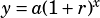Question #21, section #4, SAT test #3?

This question asks about exponential growth. In exponential growth, an initial amount is multiplied, over and over again, by a growth factor. Most savings accounts you could go to a bank today and get will provide exponential growth because they pay interest on the amount in your account when the calculate interest, rather than just paying you a flat amount each year. So each successive period, you get paid the same interest rate on slightly more money than you did last time.

To see why only choice C provides exponential growth, let’s say I have \$100 and I invest in each of the accounts described in the answer choices.

In choice A, 2% of the initial value is added each year. 2% of \$100 is \$2, so I basically get \$2 per year, forever. That’s linear growth.

In choice B, 1.5% of the initial value and an extra \$100 is added each year. That’s a great deal for me only investing \$100 up front, but it’s still not exponential growth: I get \$1.50 + \$100 = \$101.50 added per year—the same value added to my account each year, forever. That’s linear growth.

In choice C, 1% of the CURRENT VALUE is added each year. So the first year, I get \$1, which gives me \$101. The second year, I’ll get \$1.01, because that’s 1% of \$101. Then I’ll have \$102.01. The year after that, I’ll get slightly more again. Each year, I get ever so slightly more. I’m not getting rich quick with this deal, but it is exponential growth.

In choice D, again, I’m just getting the same amount added every year. That’s linear growth.

(The general equation you should have in your mind when you’re dealing with exponential growth is, where y is the current value of whatever is growing, a is its initial value, r is its growth rate in decimal form, and x is the number of periods of compounding. The formula can get more complicated than that, but probably won’t on the SAT.)